## Evolute

An evolute is the locus of centers of curvature (the envelope) of a plane curve's normals. The original curve is then said to be the Involute of its evolute. Given a plane curve represented parametrically by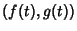, the equation of the evolute is given by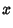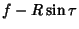(1)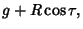(2)

where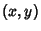are the coordinates of the running point,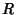is the Radius of Curvature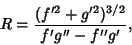(3)

and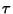is the angle between the unit Tangent Vector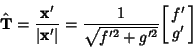(4)

and the x-Axis,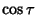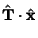(5)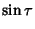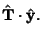(6)

Combining gives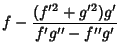(7)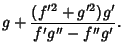(8)

The definition of the evolute of a curve is independent of parameterization for any differentiable function (Gray 1993). If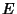is the evolute of a curve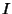, thenis said to be the Involute of. The centers of the Osculating Circles to a curve form the evolute to that curve (Gray 1993, p. 90).

The following table lists the evolutes of some common curves.

 Curve Evolute Astroid Astroid 2 times as large Cardioid Cardioid 1/3 as large Cayley's Sextic Nephroid Circle point (0, 0) Cycloid equal Cycloid Deltoid Deltoid 3 times as large Ellipse Lamé Curve Epicycloid enlarged Epicycloid Hypocycloid similar Hypocycloid Limaçon Circle Catacaustic for a point source Logarithmic Spiral equal Logarithmic Spiral Nephroid Nephroid 1/2 as large Parabola Neile's Parabola Tractrix Catenary

References

Cayley, A. On Evolutes of Parallel Curves.'' Quart. J. Pure Appl. Math. 11, 183-199, 1871.

Dixon, R. String Drawings.'' Ch. 2 in Mathographics. New York: Dover, pp. 75-78, 1991.

Gray, A. Evolutes.'' §5.1 in Modern Differential Geometry of Curves and Surfaces. Boca Raton, FL: CRC Press, pp. 76-80, 1993.

Jeffrey, H. M. On the Evolutes of Cubic Curves.'' Quart. J. Pure Appl. Math. 11, 78-81 and 145-155, 1871.

Lawrence, J. D. A Catalog of Special Plane Curves. New York: Dover, pp. 40 and 202, 1972.

Lee, X. Evolute.'' http://www.best.com/~xah/SpecialPlaneCurves_dir/Evolute_dir/evolute.html.

Lockwood, E. H. Evolutes and Involutes.'' Ch. 21 in A Book of Curves. Cambridge, England: Cambridge University Press, pp. 166-171, 1967.

Yates, R. C. Evolutes.'' A Handbook on Curves and Their Properties. Ann Arbor, MI: J. W. Edwards, pp. 86-92, 1952.